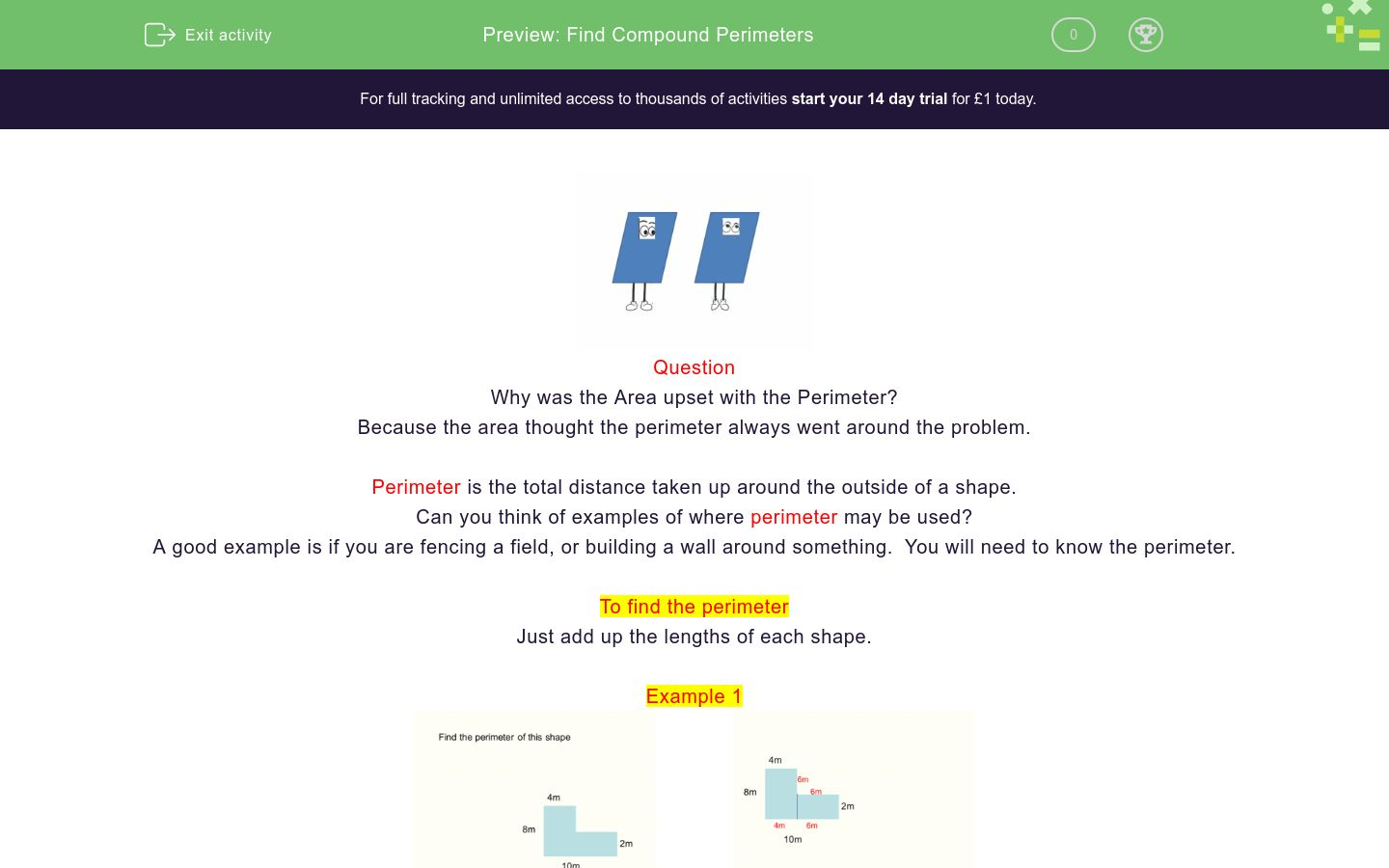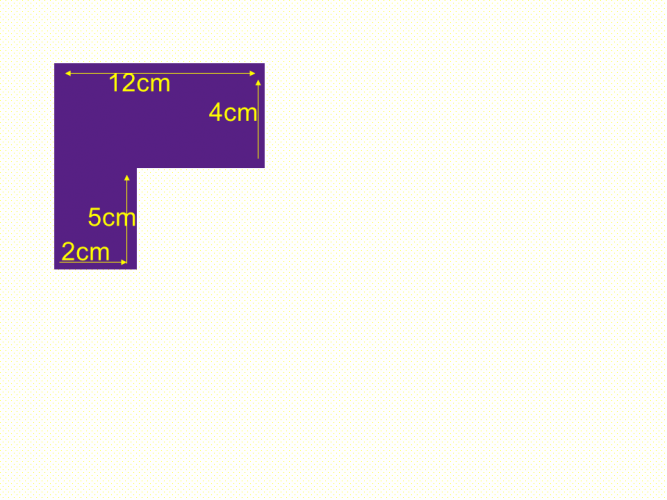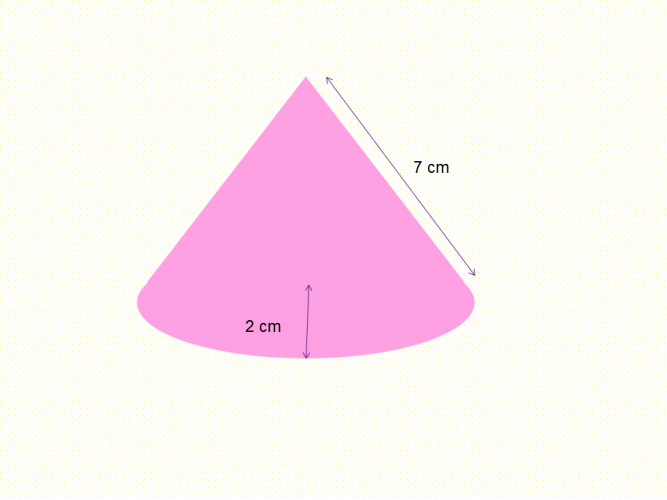# Find Compound Perimeters

In this worksheet, students will find the perimeter of compound shapes including circles.Key stage:  KS 4

GCSE Subjects:   Maths

GCSE Boards:   OCR, AQA, Eduqas, Pearson Edexcel

Curriculum topic:   Mensuration, Geometry and Measures

Curriculum subtopic:   Perimeter Calculations, Mensuration and Calculation

Difficulty level:### QUESTION 1 of 10Question

Why was the Area upset with the Perimeter?

Because the area thought the perimeter always went around the problem.

Perimeter is the total distance taken up around the outside of a shape.

Can you think of examples of where perimeter may be used?

A good example is if you are fencing a field, or building a wall around something.  You will need to know the perimeter.

To find the perimeter

Just add up the lengths of each shape.

Example 1When you have a missing length such as in this example, break it down as shown above.

Perimeter = 10 + 2 + 6 + 6 + 4 + 8 = 36 cm.

Example 2Calculate the missing length.  If the slant height isn't given you have to assume the slant height is 4 cm.

Perimeter = 4 + 10 + 10 = 24 cm

Example 3The missing side length is 11 cm and the length of the semi circle can be calculated by finding the circumference and dividing by 2.

Circumference = π x diameter

The missing length of the rectangle is 11 cm.

The circumference of the circle is π x 12 = 37.70 cm

12 + 11 + 11 + 37.70 cm = 71.70 cm

Lets get around these problems.Calculate the perimeter of this shape.Find the perimeter of this shape.

40 cm

38 cm

36 cm

42 cm40 cm

38 cm

36 cm

42 cmThe radius of this circle is 6 cm.

Calculate the perimeter.

 18.84 cm 9.43 cm 37.70 cm 21.42 cm The Perimeter is18.84 cm 9.43 cm 37.70 cm 21.42 cm The Perimeter is68 cm 60 cm 54 cm 48 cm The perimeter of this shape isFind the perimeter of this regular polygon.Calculate the perimeter of this shape.

20 .28 cm

25 .52 cm

30.22 cm

29.63 cmCalculate the perimeter of this shape.

The perimeter is 42 cm, 38 cm, 46 cm, 36 cmCalculate the perimeter of this shape.

• Question 1Calculate the perimeter of this shape.

34
EDDIE SAYS
Did you find the missing sides of 9 cm and 2 cm? Perimeter is 12 + 5 + 3 + 3 + 9 + 2 = 34 cm
• Question 2Find the perimeter of this shape.

42 cm
EDDIE SAYS
Did you find the missing sides of 9 cm and 10 cm? That is the key to these type of questions. Perimeter = 12 + 4 + 10 + 5 + 2 + 9 = 42 cm
• Question 3EDDIE SAYS
Just pick out the sides of the parallelogram. And around we go. Now just add them up 10 + 10 + 10 + 10 + 15 + 15 = 70 cm.
• Question 4The radius of this circle is 6 cm.

Calculate the perimeter.

 18.84 cm 9.43 cm 37.70 cm 21.42 cm The Perimeter is
EDDIE SAYS
Only given the radius..what do I do? Find the diameter by doubling the radius. Find the circumference of the circle π x 12 = 37.70 cm Now divide by 4 to get the arc length = 9.42 cm This only gives us the arc length so we add the other two sides on. 9.42 + 6 + 6 = 21.42 cm.
• Question 5EDDIE SAYS
It is not really that awkward, maybe a little time consuming because you have to find the missing sides in particular the circle part Find the missing sides first. The opposite side of the trapezium is 7 cm. The circumference of the circle is found by using π x 8cm ÷ 4 = 6.28 cm. (8 is the diameter of the circle) Total perimeter is 6.28 cm + 4 cm + 6 cm + 7 cm + 14 cm + 7 cm + 4 cm = 48.28 cm.
• Question 668 cm 60 cm 54 cm 48 cm The perimeter of this shape is
EDDIE SAYS
It is one of these shapes where all the measurements are there, but it is just a case of making sure we don't miss any. Best thing to do is to write them down on the shape. All the opposite sides are going to be either 9 cm or 4 cm. Total perimeter if you haven't missed any out is 68 cm.
• Question 7Find the perimeter of this regular polygon.

41
EDDIE SAYS
Are you getting used to filling in the missing sides. As the shape is a regular polygon you know the missing lengths are the same as those opposite. Total perimeter 41 cm when all sides added up.
• Question 8Calculate the perimeter of this shape.

20 .28 cm
EDDIE SAYS
The opposite sloping side is also 7 cm. The circumference of the semi circle is π x 2 x 2 = 12.566.. ÷ 2 = 6.28 cm. Total perimeter = 6.28 cm + 7cm + 7 cm = 20.28 cm
• Question 9Calculate the perimeter of this shape.

The perimeter is 42 cm, 38 cm, 46 cm, 36 cm
EDDIE SAYS
This could be tricky as there are lots of missing sides to get. Although the measurements are on the diagram, it can be easy to miss one. If you got them all you should have got 46 cm.
• Question 10Calculate the perimeter of this shape.

29.07
EDDIE SAYS
Impossible, I hear you say. I haven\'t got one of the lengths of the triangle. It is one of those awkward questions thrown at us to test other knowledge. Here you will need knowledge of Pythagoras\' Theorem to calculate the missing length. It is always good to know this as it often sneaks into work where triangles are involved. To find the missing length of the triangle. First find the base of the triangle 8 - 2 - 1 = 5 cm. Apply the theorem 5² + 5² = 50 cm² √50cm² = 7.07 cm. The opposite side of the rectangle is 3 cm. Bingo you are now away. Adding the lengths of the shape gives us 29.07 cm.
---- OR ----

Sign up for a £1 trial so you can track and measure your child's progress on this activity.

### What is EdPlace?

We're your National Curriculum aligned online education content provider helping each child succeed in English, maths and science from year 1 to GCSE. With an EdPlace account you’ll be able to track and measure progress, helping each child achieve their best. We build confidence and attainment by personalising each child’s learning at a level that suits them.

Get started